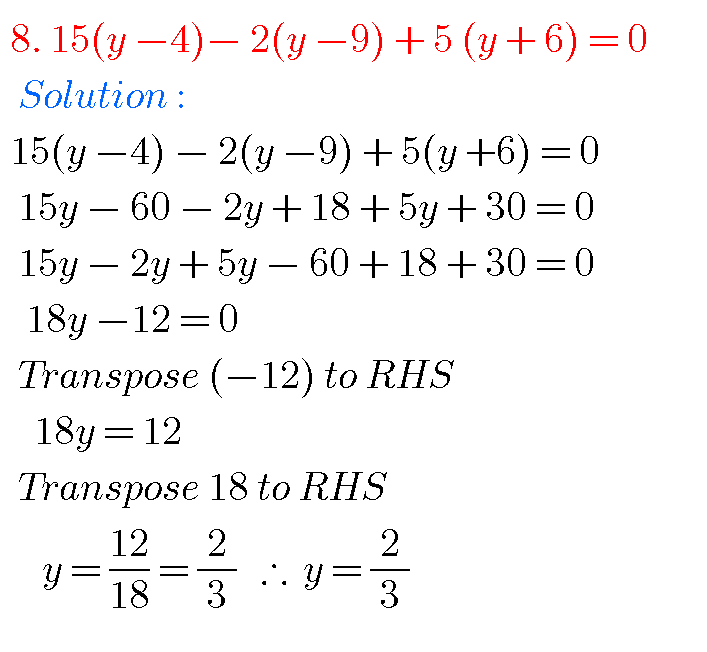## Ncert solutions for class 8 maths chapter 2,Linear Equations in One Variable solutions

NCERT SOLUTIONS FOR CLASS 8  MATHS CHAPTER 2 Class 8 Chapter 2 Linear Equations in One Variable Solutions Maths Ncert are given. First you study the textbook lesson Linear Equations in One Variable very well. Observe and practice the example problems and solutions given in the textbook. Observe the given below solutions and try them …